Math resources Geometry

3D shapes

Prism shape

# Prism shape

Here you will learn about prism shapes, including how to classify and identify prism shapes.

Students will first learn about prism shapes as a part of geometry in 1st grade and continue to explore prism shapes in 6th grade.

## What is a prism shape?

A prism shape is a 3D shape made up of flat faces, including two congruent polygonal bases and lateral sides connecting the bases. This page will cover right prisms, which have rectangular lateral faces.

For example,

Three main features of prisms are: faces, vertices and edges:

• Face – a closed, flat surface.
• Edge – connects two faces.
• Vertex (pl. vertices) – a point where three or more edges meet.

You can label the vertices (corners) of a prism to identify certain edges or faces. Below is an example of a triangular prism where the letters A-F represent the 6 vertices of the prism.

Using this labeling, you can identify lengths such as the length AB :

You can also identify faces such as the face ABC :

The number of faces, edges and vertices can vary depending on the prism. Let’s look at some examples:

ShapeFacesEdgesVertices

Triangular prism
5 9 6

Rectangular prism
6 12 8

Pentagonal prism
7 15 10

L-shaped prism
(Hexagonal prism)
8 18 12

A prism is irregular if the base is not a regular polygon.

For example, the rectangular prism and L-shaped prism are irregular prisms because their bases are irregular polygons.

### What is a prism shape?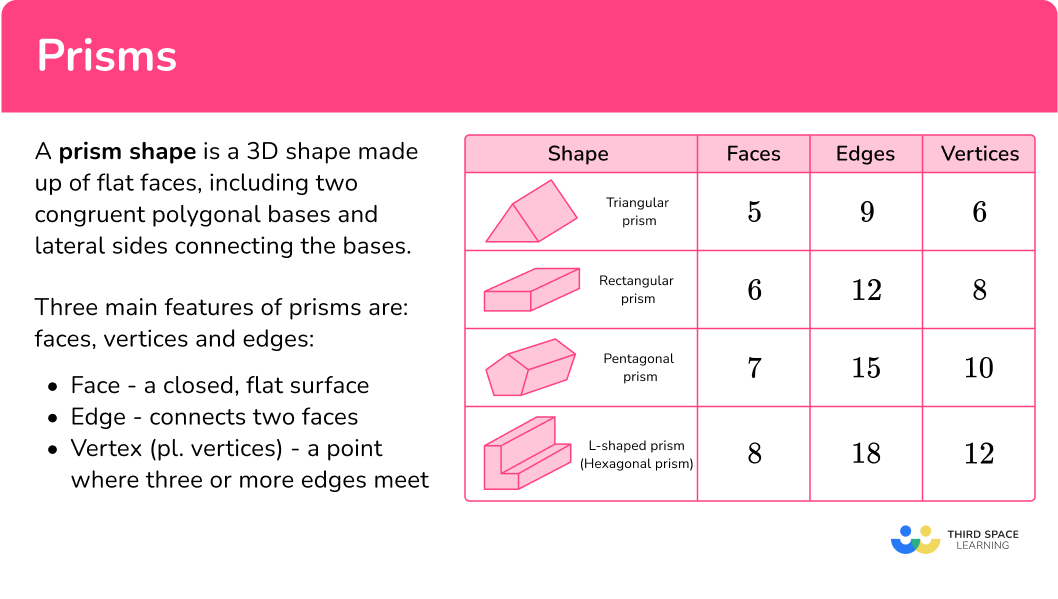## Common Core State Standards

How does this relate to 1st grade math and 6th grade math?

Compose two-dimensional shapes (rectangles, squares, trapezoids, triangles, half-circles, and quarter-circles) or three-dimensional shapes (cubes, right rectangular prisms, right circular cones, and right circular cylinders) to create a composite shape, and compose new shapes from the composite shape.

Represent three-dimensional figures using nets made up of rectangles and triangles, and use the nets to find the surface area of these figures. Apply these techniques in the context of solving real-world and mathematical problems.

## How to identify a prism shape

In order to identify a prism shape, you will:

1. Look for the characteristics of a prism.
2. State whether or not the shape is a prism.
3. If the shape is not a prism, explain what characteristics are different.

## Volume of a prism examples

### Example 1: identifying prisms

Is the shape below a prism?

1. Look for the characteristics of a prism.

The shape has 6 rectangular faces.

2State whether or not the shape is a prism.

This shape is a prism. Since the base is a rectangle, it is a rectangular prism.

### Example 2: identifying prisms

Is the shape below a prism?

The shape has a circular base and no lateral faces.

The shape is not a prism. It is a cone.

A cone is not a prism, because it does not have two identical bases that are connected by lateral faces.

### Example 3: identifying prisms

Is the shape below a prism?

The shape has 7 faces.

This shape is a prism. Since the base of the prism is a pentagon, it is a pentagonal prism.

Note: Since the base appears to be a regular pentagon, the prism would be a regular prism.

### Example 4: identifying prisms

Is the shape below a prism?

The shape has 4 faces.

The shape is not a prism. It is a square pyramid.

A square pyramid is not a prism, because it does not have two identical bases that are connected by lateral faces.

### Example 5: identifying prisms

Is the shape below a prism?

The shape has no bases or lateral sides.

The shape is not a prism. It is a sphere.

A sphere is not a prism, because it does not have two identical bases that are connected by lateral faces.

### Example 6: identifying prisms

Is the shape below a prism?

The shape has 5 faces:

2 triangle bases and 3 rectangular lateral faces.

This shape is a prism. Since the base of the prism is a triangle, it is a triangular prism.

Note: Since the base appears to be a right triangle, the prism would be a right triangular prism.

### Teaching tips for prism shapes

• Worksheets are one way to have students practice types of prisms, but real-life exposure to these shapes is crucial. This can include working with 3D math manipulatives, creating the prisms and their nets and identifying prisms in the real world.

### Easy mistakes to make

• Confusing edges and vertices
Edges are the straight lines where two faces meet, while a vertex is a point where edges intersect.
For example,

• Confusing 2D and 3D shapes
When learning the names and properties of shapes, it can be easy to confuse them. With repeated exposure and conversations around these shapes, it becomes easier to remember them.

• Forgetting that bases are equal
A prism has identical bases (or identical ends) that are connected by lateral faces. If a shape only has one base or the bases are not identical, the shape is not a prism.

• Thinking that oblique prisms are not prisms
Though they are not typically introduced until later grades, oblique prisms fit the definition and are prisms. If younger students come across these in the real world or ask questions about these shapes, this gives an opportunity to discuss why they are prisms, but how they are different from right prisms.
For example,
The oblique rectangular prism below has two identical bases and parallelogram lateral faces.

### Practice prism shape questions

1. Which shape is a prism?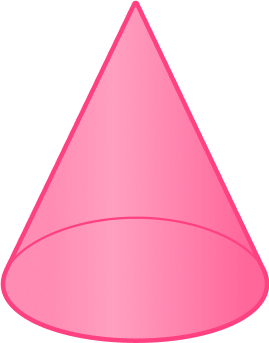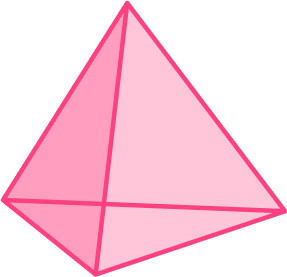This shape has two rectangular bases and four rectangular lateral faces – it is a rectangular prism.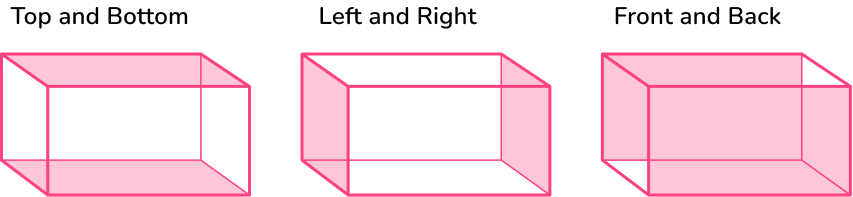2. Which shape is NOT a prism?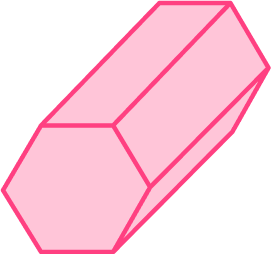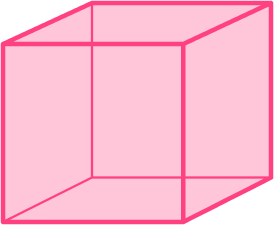This shape has two identical bases, but it does not have any lateral faces. It is not a prism – it is a cylinder.3. Which shape is a prism?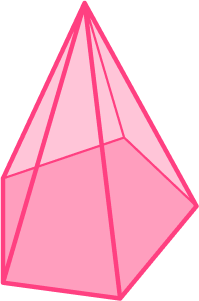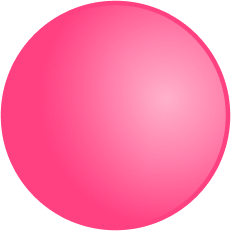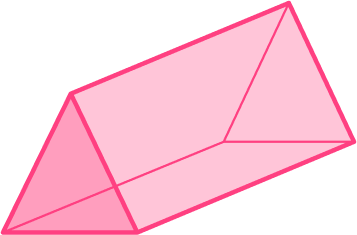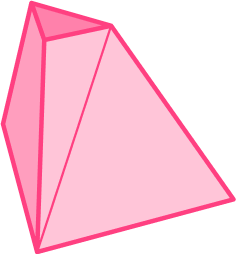This shape has two triangular bases and three rectangular lateral faces – it is a triangular prism.4. Which shape is NOT a prism?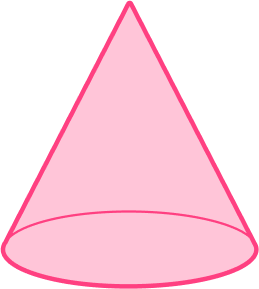This shape has one base and it does not have any lateral faces. It is not a prism – it is a cone.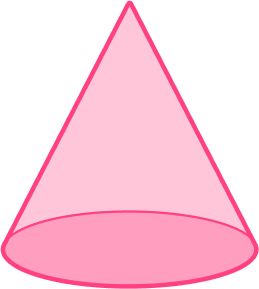5. Which shape is a triangular prism?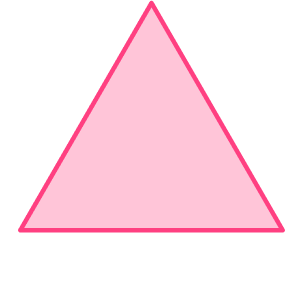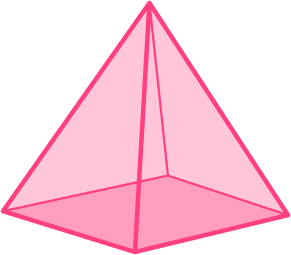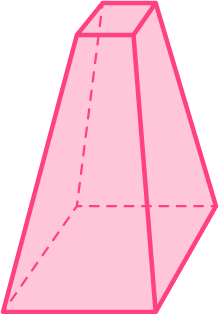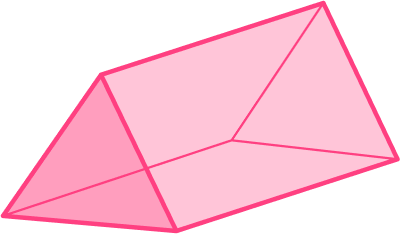This shape has two triangular bases and three rectangular lateral faces – it is a triangular prism.6. Which shape is a rectangular prism?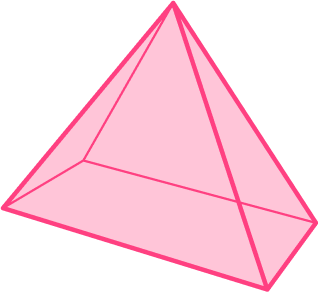This shape has two rectangular bases and four rectangular lateral faces – it is a rectangular prism.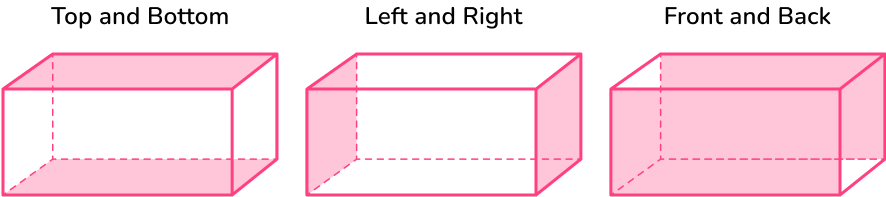## Prism shape FAQs

What is an octagonal prism?

A prism with two identical octagonal bases connected by lateral faces.

How do you calculate the volume of a rectangular prism?

Take the area of the base and multiply it by the height of the prism. Record the answer in cubic units.

What are cross-sections?

Cross-sections are the 2D shape that is created when a 3D shape is sliced.

## Still stuck?

At Third Space Learning, we specialize in helping teachers and school leaders to provide personalized math support for more of their students through high-quality, online one-on-one math tutoring delivered by subject experts.

Each week, our tutors support thousands of students who are at risk of not meeting their grade-level expectations, and help accelerate their progress and boost their confidence.